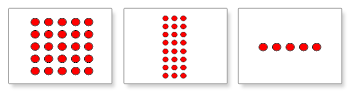# More About Each Lesson Type

Before you start the lessons with your child, it is useful to know a bit more about the different lesson types, how they work, and how they are supposed to be played.

Quantity Lesson

With lessons on quantity, you can help your child understand the reality of numbers (instead of just the symbols - 1, 2, etc. - that represent them). This will give your child a deeper understanding of mathematical concepts. These lessons use icons to illustrate real quantities and form the foundation of the math lessons to follow.A variation of Quantity Lesson is Multiples Lesson. Similar to lessons on quantity, Little Math also uses icons to present the concept of multiples to your child.Numeral Lesson

Little Math also supports teaching math using written numerals, so your child can learn to recognize the written representations of the number quantities. Numerals are introduced once the foundation in quantity, and equations that use quantity, has been established. A week after your child begins learning numerals, equations will begin using numerals as well as quantities. Now, when a symbol (numeral) is shown, your child will readily associate it with the reality – the quantity.Math Lesson (Equations)

Little Math uses icons and numeric symbols of the numbers to teach mathematical operations. Depending on your child's level of learning, you can teach simple two-step equations, and advance to using up to 5 integers for operations. By using real quantities in equations, we can make addition, subtraction, multiplication and division instantly comprehensible, even to very young children. Appearing as they really are - as quantities - the numbers engage the child's logical thinking.Related Article:

Lesson Panel